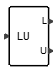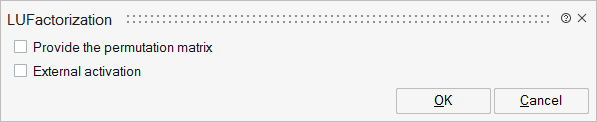# LUFactorization

This block outputs two matrices L and U, with row pivoting, from the LU factorization of a square input matrix. If A is the input matrix then E*A=L*U where E is a permutation matrix.MatrixOperations

## Description

The LU block performs the LU factorization of a matrix A. The matrix A must be a square matrix. The output is two matrices L and U, with row pivoting.

The factorization is such that E*A=L*U where A is the input square matrix, E is the permutation matrix, U an upper triangular matrix and L a lower triangular matrix.

## ParametersNameLabelDescriptionData TypeValid Values

plu

Provide the permutation matrix

If this paramater is turned ON, the block has one additional output port to provide the permutation matrix.

Number

0
1

externalActivation

External activation

Specifies whether the block receives an external activation or inherits its activation through its regular input ports. When External Activation is selected, an additional activation port is added to the block. By default, external activation is not selected.

Number

0
1

## Ports

NameTypeDescriptionIO TypeNumber

Port 1

explicit

Input Matrix A (matrix to be factorized)

input

1

L

explicit

output

1

U

explicit

output

2

Port 4

explicit

output

plu

Port 5

activation

External activation

input

externalActivation

NameValueDescription

always active

no

direct-feedthrough

yes

zero-crossing

no

mode

no

continuous-time state

no

discrete-time state

no